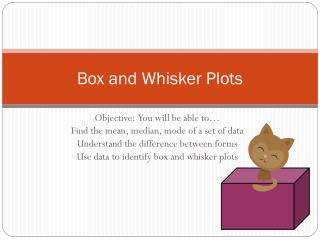DownloadDownload PresentationBox and Whisker Plots

# Box and Whisker Plots

Télécharger la présentation## Box and Whisker Plots

- - - - - - - - - - - - - - - - - - - - - - - - - - - E N D - - - - - - - - - - - - - - - - - - - - - - - - - - -
##### Presentation Transcript

1. Box and Whisker Plots Objective: You will be able to… Find the mean, median, mode of a set of data Understand the difference between forms Use data to identify box and whisker plots

2. Box-and-Whisker Plot Practice There are 12 people are in a Habanera Pepper eating contest who ate the following amount of peppers: 4, 4, 4, 9, 15, 2, 5, 0, 10, 12, 1, 18 Put the numbers in order from least to greatest: 0, 1, 2, 4, 4, 4, 5, 9, 10, 12, 15, 18 BELOW THE MEDIAN (4.5) ABOVE THE MEDIAN (4.5) Discussion Question: Why are all of the Boxes and Whiskers different sizes?

3. _____% _____% _____% _____% Analyzing a Box-and-Whisker Plot 25% 25% 25% 25% 50% 50%

4. Box-and-Whisker Plot Reflection Question • Nate believes that the reason that the boxes and whiskers are not always the same size is because there are more numbers in some of the boxes than others. Is he correct? Explain.

5. Example using Percents • The box and Whisker plot shows that math grades of 100 students. • What percent of the grades are between 68 and 78? • What is the Lower Extreme? • What is the Interquartile Range? • What percent of students scored above 78? • Which is true? • Half of the grades are below 78 • Half of the grades are between 68 and 78 • The average grade is 78 • There were more grades between 78 and 86 than between 67 and 68

6. Example Stem and Leaf Plot • Stem and Leaf Plots- • a way of displaying numbers • Rewrite the numbers from least to greatest • Order: 12 , 13 , • Lower Extreme: • Lower Quartile: • Median: • Upper Quartile: • Upper Extreme: • Range: • IQR:

7. You TRY! • Order: • Lower Extreme: • Lower Quartile: • Median: • Upper Quartile: • Upper Extreme: • Range: • IQR:

8. Example 1

9. Example 2 The are 11 people at a school with their monthly salaries displayed below: • Exactly 2 people earn \$5,000 or more each month • Exactly 5 people earn less than \$5,000 each month • Exactly 5 people earn \$4,000 or less each month • Exactly 6 people earn \$4,000 or more each month

10. Example 3 The are 8 people at a school with their monthly salaries displayed below: • Exactly 4 people earn \$5,000 or more each month • Exactly 5 people earn less than \$5,000 each month • Exactly 4 people earn \$4,000 or less each month • Exactly 5 people earn \$4,000 or more each month

11. Example 4

12. Colby graphed some data as shown in this box-and-whisker plot. • The range of the data is 25. • Half of the data is below 65. • The median of the data is 60. • Three-fourths of the data is below 90. Which statement is true about Colby’s data? Bellwork: Thursday